Скачать презентацию Chapter 8 Logistic Regression 1 Introduction

affc55fa96f0bdff35db1760705a9f3a.ppt

• Количество слайдов: 13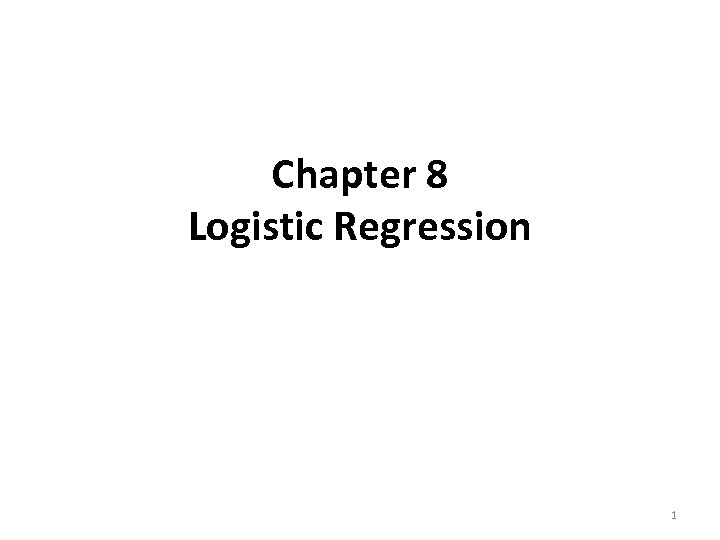Chapter 8 Logistic Regression 1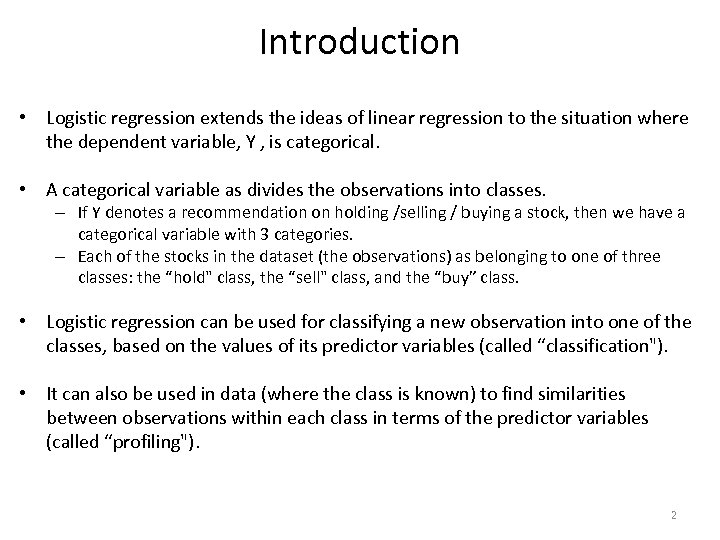Introduction • Logistic regression extends the ideas of linear regression to the situation where the dependent variable, Y , is categorical. • A categorical variable as divides the observations into classes. – If Y denotes a recommendation on holding /selling / buying a stock, then we have a categorical variable with 3 categories. – Each of the stocks in the dataset (the observations) as belonging to one of three classes: the “hold" class, the “sell" class, and the “buy” class. • Logistic regression can be used for classifying a new observation into one of the classes, based on the values of its predictor variables (called “classification"). • It can also be used in data (where the class is known) to find similarities between observations within each class in terms of the predictor variables (called “profiling"). 2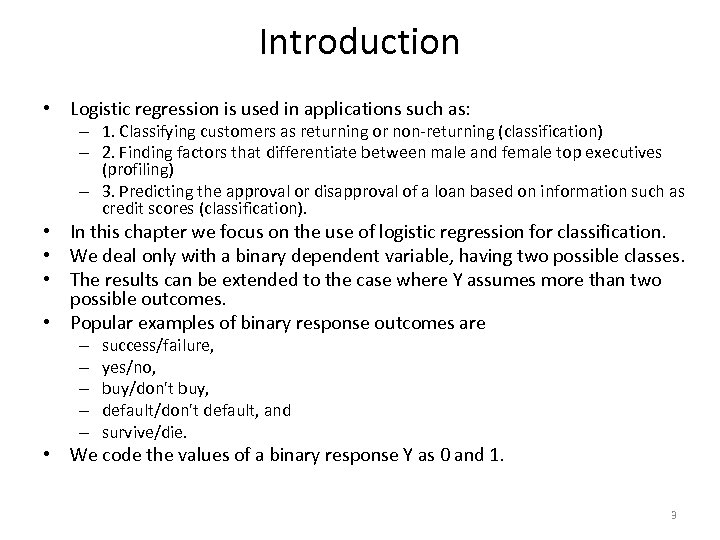Introduction • Logistic regression is used in applications such as: – 1. Classifying customers as returning or non-returning (classification) – 2. Finding factors that differentiate between male and female top executives (profiling) – 3. Predicting the approval or disapproval of a loan based on information such as credit scores (classification). • In this chapter we focus on the use of logistic regression for classification. • We deal only with a binary dependent variable, having two possible classes. • The results can be extended to the case where Y assumes more than two possible outcomes. • Popular examples of binary response outcomes are – – – success/failure, yes/no, buy/don't buy, default/don't default, and survive/die. • We code the values of a binary response Y as 0 and 1. 3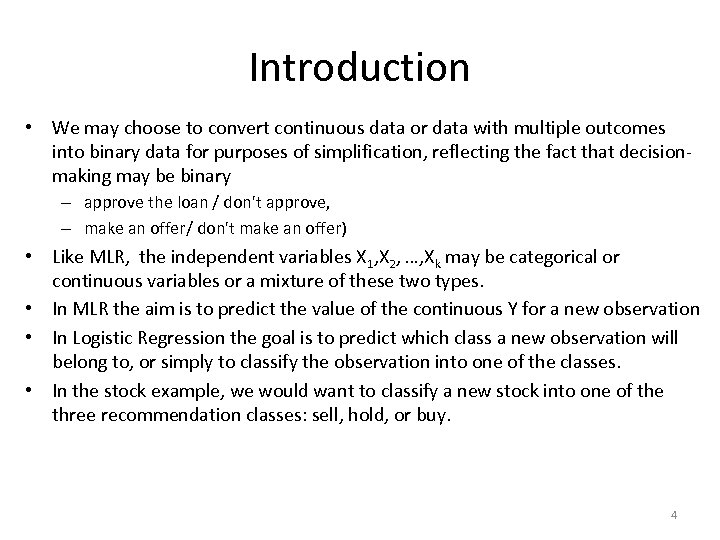Introduction • We may choose to convert continuous data or data with multiple outcomes into binary data for purposes of simplification, reflecting the fact that decisionmaking may be binary – approve the loan / don't approve, – make an offer/ don't make an offer) • Like MLR, the independent variables X 1, X 2, …, Xk may be categorical or continuous variables or a mixture of these two types. • In MLR the aim is to predict the value of the continuous Y for a new observation • In Logistic Regression the goal is to predict which class a new observation will belong to, or simply to classify the observation into one of the classes. • In the stock example, we would want to classify a new stock into one of the three recommendation classes: sell, hold, or buy. 4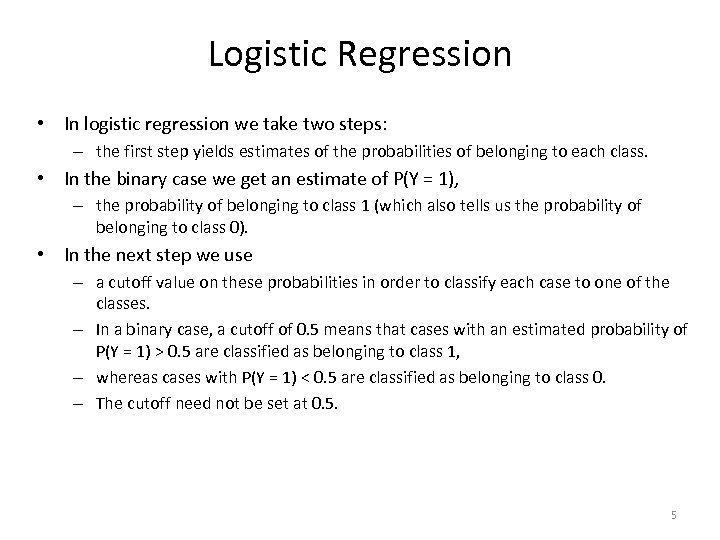Logistic Regression • In logistic regression we take two steps: – the first step yields estimates of the probabilities of belonging to each class. • In the binary case we get an estimate of P(Y = 1), – the probability of belonging to class 1 (which also tells us the probability of belonging to class 0). • In the next step we use – a cutoff value on these probabilities in order to classify each case to one of the classes. – In a binary case, a cutoff of 0. 5 means that cases with an estimated probability of P(Y = 1) > 0. 5 are classified as belonging to class 1, – whereas cases with P(Y = 1) < 0. 5 are classified as belonging to class 0. – The cutoff need not be set at 0. 5. 5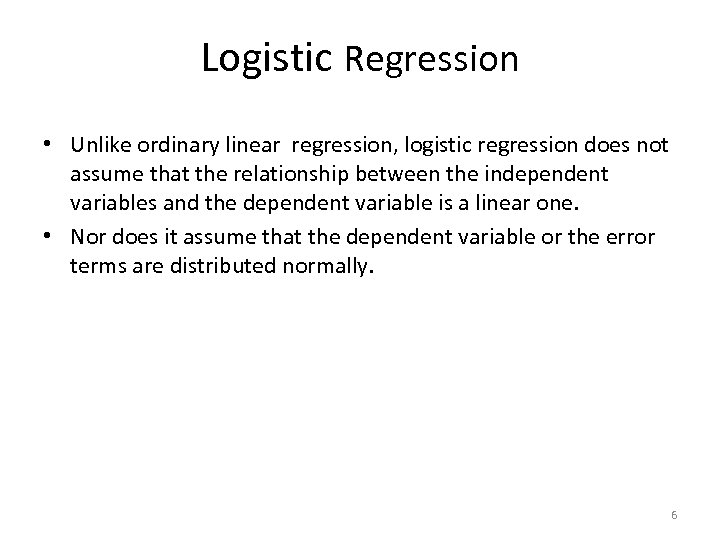Logistic Regression • Unlike ordinary linear regression, logistic regression does not assume that the relationship between the independent variables and the dependent variable is a linear one. • Nor does it assume that the dependent variable or the error terms are distributed normally. 6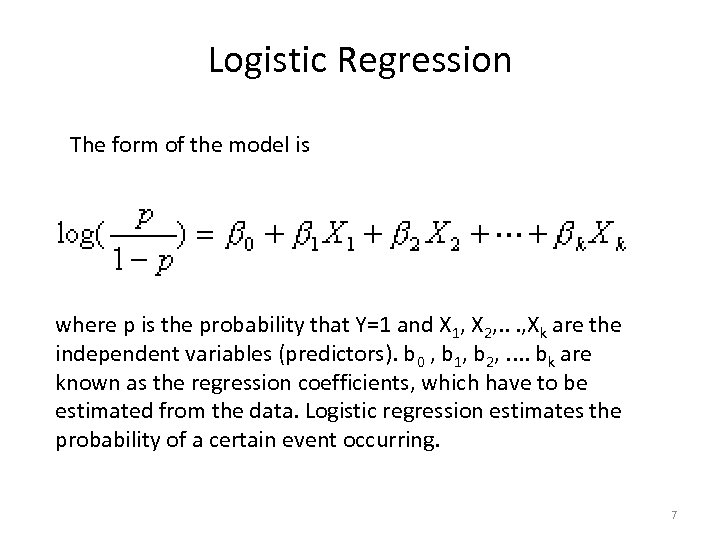Logistic Regression The form of the model is where p is the probability that Y=1 and X 1, X 2, . . . , Xk are the independent variables (predictors). b 0 , b 1, b 2, . . bk are known as the regression coefficients, which have to be estimated from the data. Logistic regression estimates the probability of a certain event occurring. 7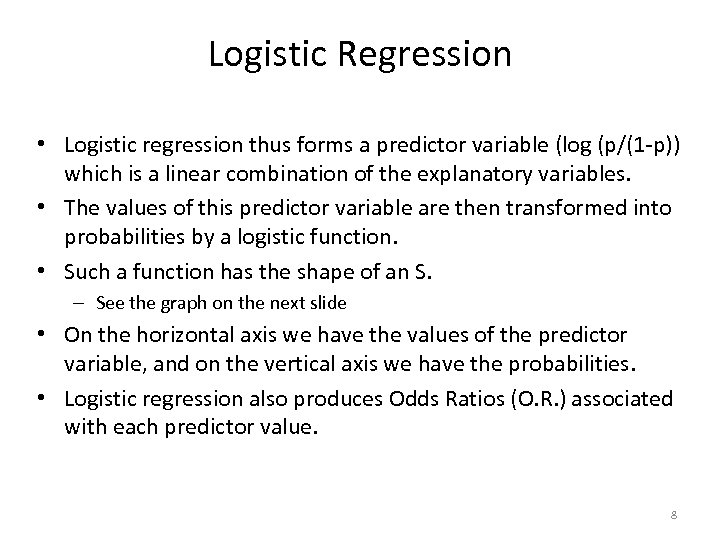Logistic Regression • Logistic regression thus forms a predictor variable (log (p/(1 -p)) which is a linear combination of the explanatory variables. • The values of this predictor variable are then transformed into probabilities by a logistic function. • Such a function has the shape of an S. – See the graph on the next slide • On the horizontal axis we have the values of the predictor variable, and on the vertical axis we have the probabilities. • Logistic regression also produces Odds Ratios (O. R. ) associated with each predictor value. 8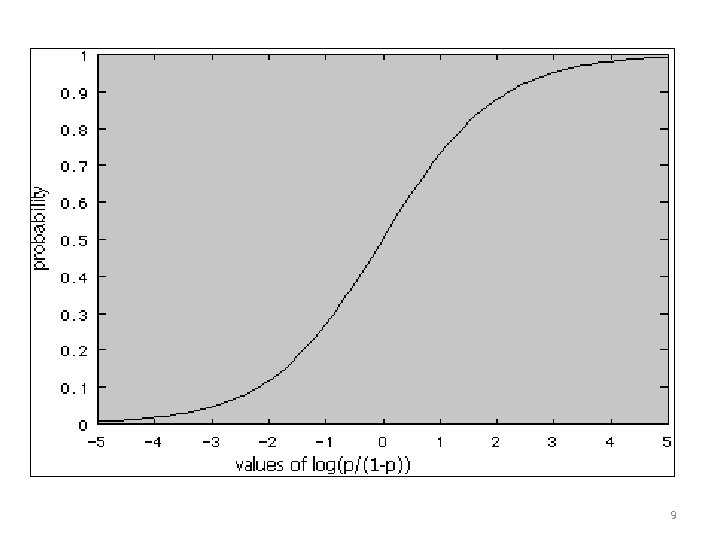9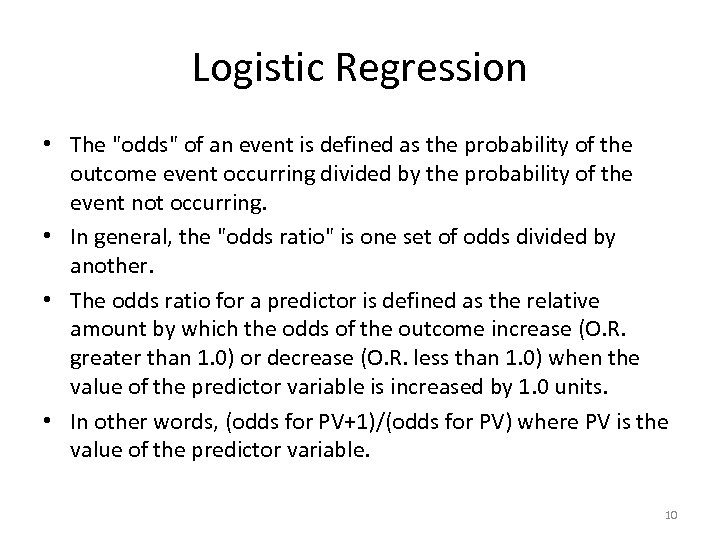Logistic Regression • The "odds" of an event is defined as the probability of the outcome event occurring divided by the probability of the event not occurring. • In general, the "odds ratio" is one set of odds divided by another. • The odds ratio for a predictor is defined as the relative amount by which the odds of the outcome increase (O. R. greater than 1. 0) or decrease (O. R. less than 1. 0) when the value of the predictor variable is increased by 1. 0 units. • In other words, (odds for PV+1)/(odds for PV) where PV is the value of the predictor variable. 10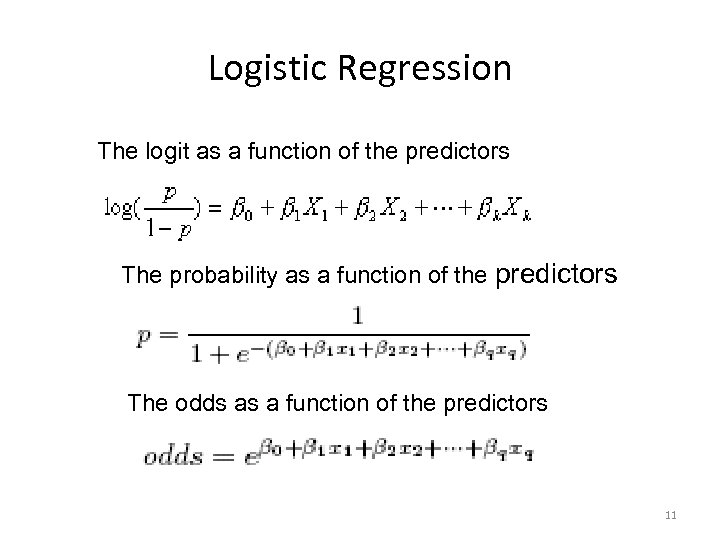Logistic Regression The logit as a function of the predictors The probability as a function of the predictors The odds as a function of the predictors 11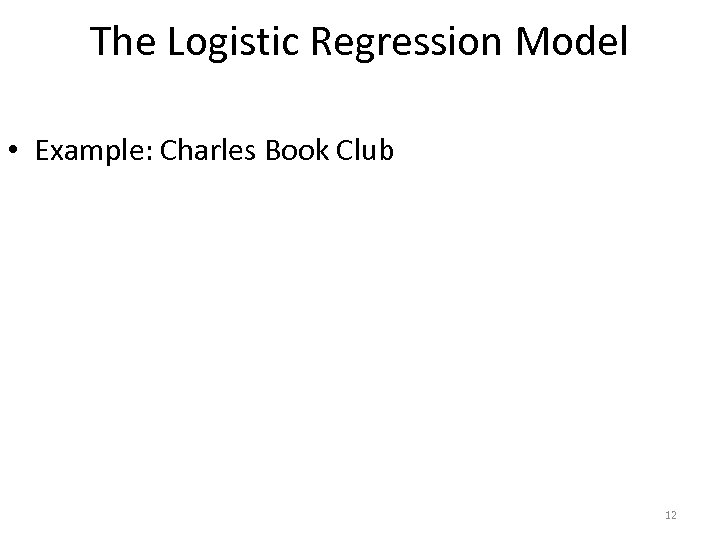The Logistic Regression Model • Example: Charles Book Club 12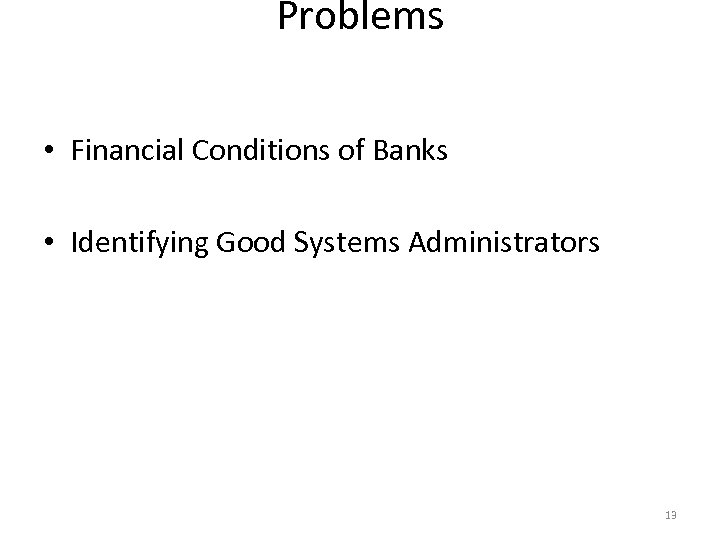Problems • Financial Conditions of Banks • Identifying Good Systems Administrators 13